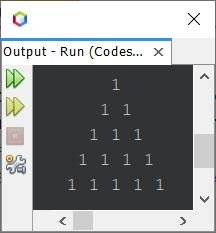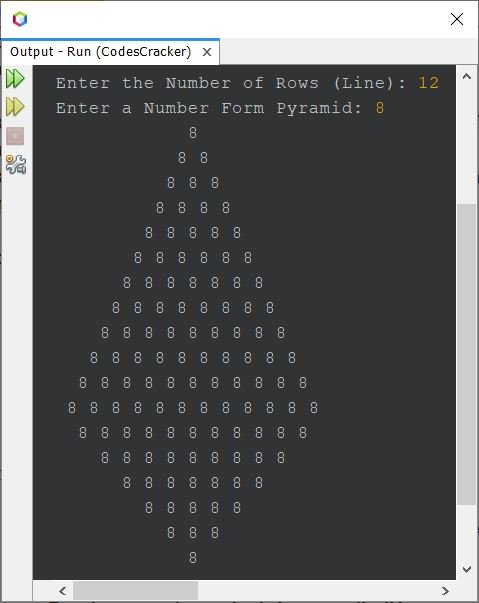# Java Program to Print Pyramid Pattern of Numbers

## Pyramid Pattern of Numbers in Java

The question is, write a Java program to print pyramid of number. Following program is its answer:

```public class CodesCracker
{
public static void main(String[] args)
{
int i, space, j, num=1;
for(i=0; i<5; i++)
{
for(space=i; space<5; space++)
System.out.print(" ");
for(j=0; j<(i+1); j++)
System.out.print(num+ " ");
System.out.print("\n");
}
}
}```

The snapshot given below shows the sample output produced by above Java program:Now let's modify the above program, to allow user to define the size of pyramid, along with the number to form the same pyramid, of given size, with given number:

```import java.util.Scanner;

public class CodesCracker
{
public static void main(String[] args)
{
int tot, i, space, j, num;
Scanner s = new Scanner(System.in);

System.out.print("Enter the Number of Rows (Line): ");
tot = s.nextInt();
System.out.print("Enter the Number to Form Pyramid: ");
num = s.nextInt();

for(i=0; i<tot; i++)
{
for(space=i; space<tot; space++)
System.out.print(" ");
for(j=0; j<(i+1); j++)
System.out.print(num+ " ");
System.out.print("\n");
}
}
}```

The sample run of above program with user input 11 as number of lines and 5 as number to form pyramid that are of 11 rows or lines, is shown in the snapshot given below:## Full Pyramid Pattern of Number in Java

This is the last program of this article, created to print the full pyramid pattern using a given number. The pyramid printed using this program, provides the actual look of pyramid.

```import java.util.Scanner;

public class CodesCracker
{
public static void main(String[] args)
{
int i, row, k, j, num;
Scanner s = new Scanner(System.in);

System.out.print("Enter the Number of Rows (Line): ");
row = s.nextInt();
System.out.print("Enter a Number Form Pyramid: ");
num = s.nextInt();

for(i=0; i<row; i++)
{
for(k=i; k<row; k++)
System.out.print(" ");
for(j=0; j<(i+1); j++)
System.out.print(num+ " ");
System.out.print("\n");
}
for(i=row; i>0; i=(i-2))
{
for(k=row; k>=(i-1); k--)
System.out.print(" ");
for(j=(i-1); j>0; j--)
System.out.print(num+ " ");
System.out.print("\n");
}
}
}```

The snapshot given below shows the sample run of above program with user input 12 as number of lines and 8 as number to form complete pyramid:Note - For more pyramid pattern using number, refer to Pyramid Pattern of Stars in Java. The only change to do, is replace the star with desired number.

Java Online Test

« Previous Program Next Program »